# Robust music signal separation based on supervised nonnegative matrix factorization with prevention of basis sharing

Mar. 18, 2015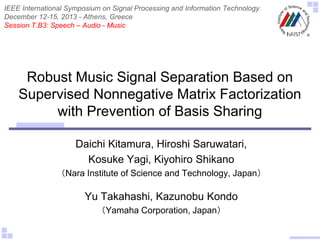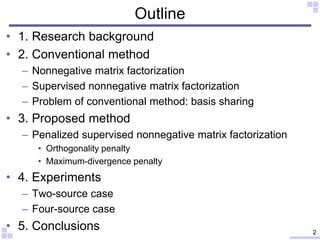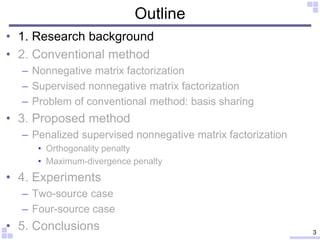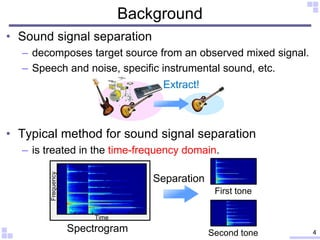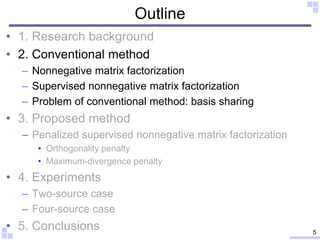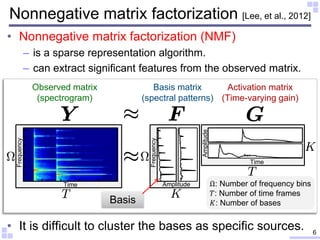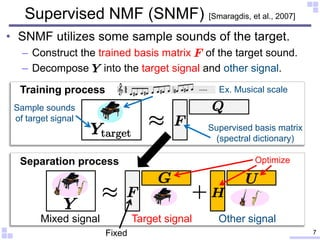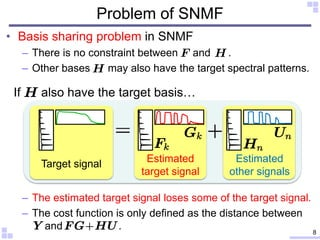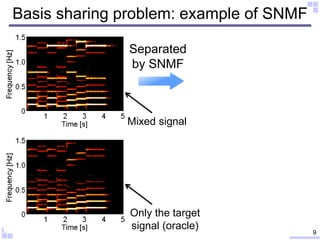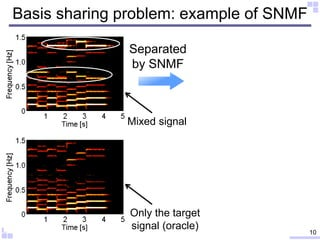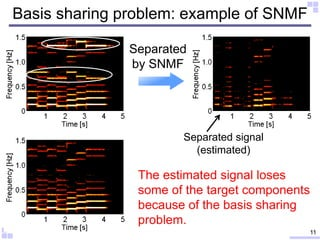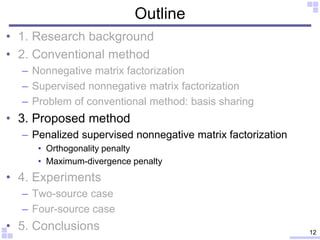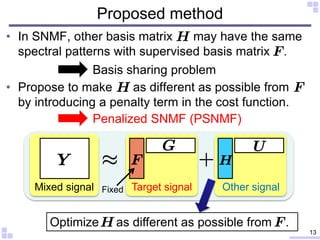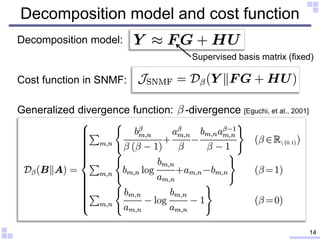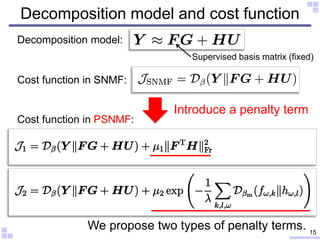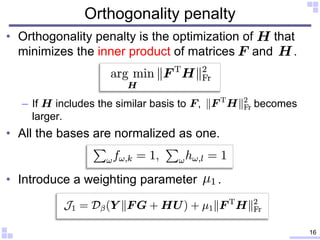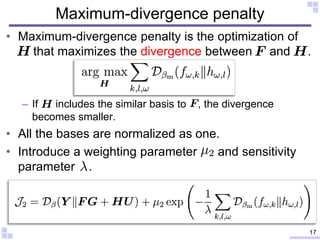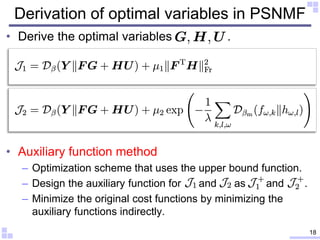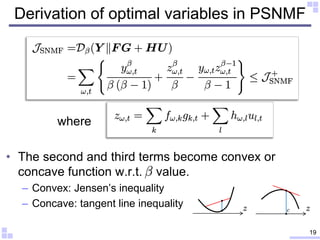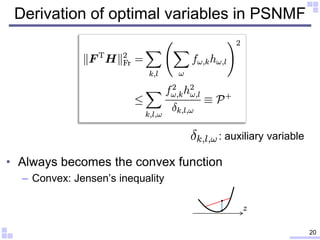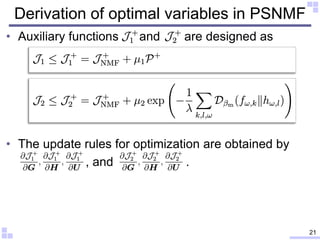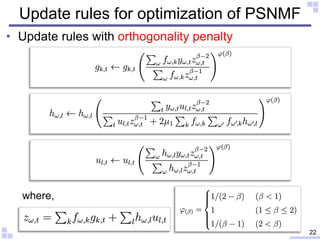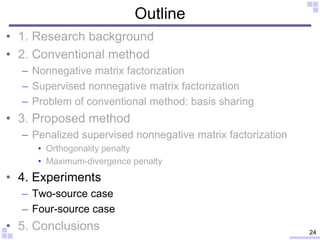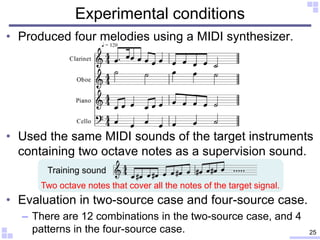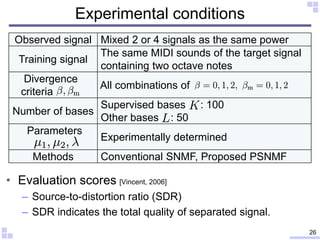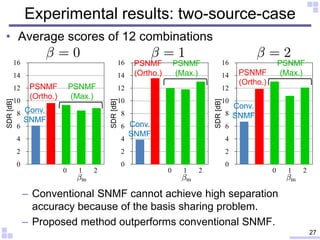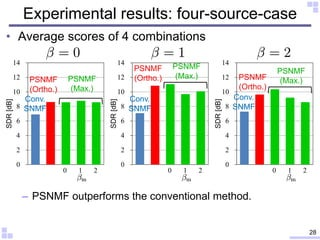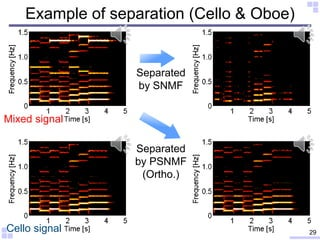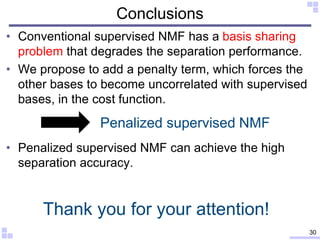1 of 30

### Robust music signal separation based on supervised nonnegative matrix factorization with prevention of basis sharing

1. Robust Music Signal Separation Based on Supervised Nonnegative Matrix Factorization with Prevention of Basis Sharing Daichi Kitamura, Hiroshi Saruwatari, Kosuke Yagi, Kiyohiro Shikano （Nara Institute of Science and Technology, Japan） Yu Takahashi, Kazunobu Kondo （Yamaha Corporation, Japan） IEEE International Symposium on Signal Processing and Information Technology December 12-15, 2013 - Athens, Greece Session T.B3: Speech – Audio - Music
2. Outline • 1. Research background • 2. Conventional method – Nonnegative matrix factorization – Supervised nonnegative matrix factorization – Problem of conventional method: basis sharing • 3. Proposed method – Penalized supervised nonnegative matrix factorization • Orthogonality penalty • Maximum-divergence penalty • 4. Experiments – Two-source case – Four-source case • 5. Conclusions 2
3. Outline • 1. Research background • 2. Conventional method – Nonnegative matrix factorization – Supervised nonnegative matrix factorization – Problem of conventional method: basis sharing • 3. Proposed method – Penalized supervised nonnegative matrix factorization • Orthogonality penalty • Maximum-divergence penalty • 4. Experiments – Two-source case – Four-source case • 5. Conclusions 3
4. • Sound signal separation – decomposes target source from an observed mixed signal. – Speech and noise, specific instrumental sound, etc. • Typical method for sound signal separation – is treated in the time-frequency domain. Background Extract! Time Frequency Spectrogram First tone Second tone Separation 4
5. Outline • 1. Research background • 2. Conventional method – Nonnegative matrix factorization – Supervised nonnegative matrix factorization – Problem of conventional method: basis sharing • 3. Proposed method – Penalized supervised nonnegative matrix factorization • Orthogonality penalty • Maximum-divergence penalty • 4. Experiments – Two-source case – Four-source case • 5. Conclusions 5
6. • Nonnegative matrix factorization (NMF) – is a sparse representation algorithm. – can extract significant features from the observed matrix. • It is difficult to cluster the bases as specific sources. Nonnegative matrix factorization [Lee, et al., 2012] Amplitude Amplitude Observed matrix (spectrogram) Basis matrix (spectral patterns) Activation matrix (Time-varying gain) Time Ω: Number of frequency bins 𝑇: Number of time frames 𝐾: Number of bases Time Frequency Frequency 6 Basis
7. • SNMF utilizes some sample sounds of the target. – Construct the trained basis matrix of the target sound. – Decompose into the target signal and other signal. Supervised NMF (SNMF) [Smaragdis, et al., 2007] Separation process Optimize Training process Supervised basis matrix (spectral dictionary) Sample sounds of target signal 7Fixed Ex. Musical scale Target signal Other signalMixed signal
8. Problem of SNMF • Basis sharing problem in SNMF – There is no constraint between and . – Other bases may also have the target spectral patterns. – The estimated target signal loses some of the target signal. – The cost function is only defined as the distance between 8 Estimated target signal Estimated other signals Target signal If also have the target basis… and .
9. Basis sharing problem: example of SNMF 9 Separated by SNMF Mixed signal Only the target signal (oracle)
10. Basis sharing problem: example of SNMF 10 Only the target signal (oracle) Separated by SNMF Mixed signal
11. Basis sharing problem: example of SNMF 11 Separated by SNMF Separated signal (estimated) The estimated signal loses some of the target components because of the basis sharing problem.
12. Outline • 1. Research background • 2. Conventional method – Nonnegative matrix factorization – Supervised nonnegative matrix factorization – Problem of conventional method: basis sharing • 3. Proposed method – Penalized supervised nonnegative matrix factorization • Orthogonality penalty • Maximum-divergence penalty • 4. Experiments – Two-source case – Four-source case • 5. Conclusions 12
13. Proposed method • In SNMF, other basis matrix may have the same spectral patterns with supervised basis matrix . • Propose to make as different as possible from by introducing a penalty term in the cost function. 13 Target signal Other signalMixed signal Fixed Optimize as different as possible from . Basis sharing problem Penalized SNMF (PSNMF)
14. Decomposition model and cost function 14 Decomposition model: Cost function in SNMF: Generalized divergence function: -divergence [Eguchi, et al., 2001] Supervised basis matrix (fixed)
15. Decomposition model and cost function 15 Introduce a penalty term We propose two types of penalty terms. Cost function in PSNMF: Decomposition model: Cost function in SNMF: Supervised basis matrix (fixed)
16. Orthogonality penalty • Orthogonality penalty is the optimization of that minimizes the inner product of matrices and . – If includes the similar basis to , becomes larger. • All the bases are normalized as one. • Introduce a weighting parameter . 16
17. Maximum-divergence penalty • Maximum-divergence penalty is the optimization of – If includes the similar basis to , the divergence becomes smaller. • All the bases are normalized as one. • Introduce a weighting parameter and sensitivity parameter . 17 that maximizes the divergence between and .
18. Derivation of optimal variables in PSNMF • Derive the optimal variables . • Auxiliary function method – Optimization scheme that uses the upper bound function. – Design the auxiliary function for and as and . – Minimize the original cost functions by minimizing the auxiliary functions indirectly. 18
19. Derivation of optimal variables in PSNMF • The second and third terms become convex or concave function w.r.t. value. – Convex: Jensen’s inequality – Concave: tangent line inequality 19 where
20. Derivation of optimal variables in PSNMF • Always becomes the convex function – Convex: Jensen’s inequality 20 : auxiliary variable
21. Derivation of optimal variables in PSNMF • Auxiliary functions and are designed as • The update rules for optimization are obtained by 21 , and .
22. Update rules for optimization of PSNMF • Update rules with orthogonality penalty 22 where,
23. Update rules for optimization of PSNMF • Update rules with maximum-divergence penalty 23 where,
24. Outline • 1. Research background • 2. Conventional method – Nonnegative matrix factorization – Supervised nonnegative matrix factorization – Problem of conventional method: basis sharing • 3. Proposed method – Penalized supervised nonnegative matrix factorization • Orthogonality penalty • Maximum-divergence penalty • 4. Experiments – Two-source case – Four-source case • 5. Conclusions 24
25. • Produced four melodies using a MIDI synthesizer. • Used the same MIDI sounds of the target instruments containing two octave notes as a supervision sound. • Evaluation in two-source case and four-source case. – There are 12 combinations in the two-source case, and 4 patterns in the four-source case. Experimental conditions 25 Training sound Two octave notes that cover all the notes of the target signal.
26. • Evaluation scores [Vincent, 2006] – Source-to-distortion ratio (SDR) – SDR indicates the total quality of separated signal. Experimental conditions Observed signal Mixed 2 or 4 signals as the same power Training signal The same MIDI sounds of the target signal containing two octave notes Divergence criteria All combinations of Number of bases Supervised bases : 100 Other bases : 50 Parameters Experimentally determined Methods Conventional SNMF, Proposed PSNMF 26
27. 0 2 4 6 8 10 12 14 16 SDR[dB] 0 2 4 6 8 10 12 14 16 SDR[dB] 0 2 4 6 8 10 12 14 16 SDR[dB] • Average scores of 12 combinations – Conventional SNMF cannot achieve high separation accuracy because of the basis sharing problem. – Proposed method outperforms conventional SNMF. Experimental results: two-source-case 27 Conv. SNMF PSNMF (Ortho.) PSNMF (Max.) PSNMF (Ortho.) PSNMF (Max.) PSNMF (Ortho.) PSNMF (Max.) 0 1 2 0 1 2 0 1 2 Conv. SNMF Conv. SNMF
28. • Average scores of 4 combinations – PSNMF outperforms the conventional method. 0 2 4 6 8 10 12 14 SDR[dB] 0 2 4 6 8 10 12 14 SDR[dB] 0 2 4 6 8 10 12 14 SDR[dB] Experimental results: four-source-case 28 PSNMF (Ortho.) PSNMF (Max.) PSNMF (Ortho.) PSNMF (Max.) PSNMF (Ortho.) PSNMF (Max.) 0 1 2 0 1 2 0 1 2 Conv. SNMF Conv. SNMF Conv. SNMF
29. Example of separation (Cello & Oboe) 29 Separated by SNMF Cello signal Mixed signal Separated by PSNMF (Ortho.)
30. Conclusions • Conventional supervised NMF has a basis sharing problem that degrades the separation performance. • We propose to add a penalty term, which forces the other bases to become uncorrelated with supervised bases, in the cost function. • Penalized supervised NMF can achieve the high separation accuracy. 30 Penalized supervised NMF Thank you for your attention!

### Editor's Notes

1. Good afternoon everyone, // I’m Daichi Kitamura from Nara institute of science and technology, Japan. Today // I’d like to talk about Robust Music Signal Separation Based on Supervised Nonnegative Matrix Factorization with Prevention of Basis Sharing.
2. This is outline of my talk.
3. First, // I talk about research background.
4. Sound signal separation / is a technique for decomposing a target signal / from an observed mixed signal. For example, / speech and noise separation, / specific instrumental sound extraction like this, / and so on. Typical method for sound signal separation is treated in the time-frequency domain, namely, in the spectrogram domain. There are two tones in this spectrogram. So, if we could separate these tones like this, / the sound separation is achieved.
5. Next, I explain about conventional methods.
6. As a means for extracting some features from the spectrogram, / nonnegative matrix factorization, NMF in short, has been proposed. This is a sparse representation algorithm, and this method can extract the significant features from the observed matrix. NMF decomposes the observed spectrogram Y, / into two nonnegative matrices F and G, approximately. (アポロークシメイトリ) Here, first decomposed matrix F has frequently-appearing spectral patterns / as a basis. And another decomposed matrix G has time-varying gains / of each spectral pattern. So, the matrix F is called as ‘basis matrix,’ / and the matrix G is called as ‘activation matrix.’ Therefore, if we could know / which basis corresponds to the target signal, we can reconstruct the target spectrogram that has only the target sound. However, it is very difficult / to cluster these bases as specific sources.
7. To solve this problem, supervised NMF, SNMF in short, has been proposed. SNMF utilizes some sample sounds of the target signal / as a supervision signal. For example, / if we wanted to separate the piano signal from this mixed signal, / the musical scale sound of the same piano / should be used as a supervision. This sample sound is decomposed by simple NMF, / and the supervised basis matrix F is constructed in the training process. Then, the mixed signal is decomposed in the separation process / using the supervised bases F, / as FG+HU. The matrix F is fixed, / and the other matrix G, H, and U are optimized. Finally, the target piano signal is separated as FG, / and the other signals, such as saxophone and bass, are separated as HU.
8. However, SNMF has a problem / called basis sharing. In SNMF, there is no constraint between the supervised matrix F and the other matrix H. Therefore, the other bases H may also have the target spectral patterns. For example, the target signal is represented as these basis and activation. The supervised matrix F has this target basis / because this is a dictionary of the target signal. If H also have this target basis, the activation is split / between G and U like this So, the target signal is deprived by HU, / and the estimated signal loses some of the target signal. This is because / the cost function is only defined as the distance between Y and FG+HU. Even if the target components are split like this, the value of cost function doesn’t change.
9. This upper left spectrogram is a mixed signal. And, lower left one is a spectrogram that have only the target signal. As you can see,
10. these components are the non-target signal. If we separate this signal using SNMF,
11. the estimated signal loses some of the target components / because of the basis sharing problem.
12. Next, I talk about our proposed method.
13. In conventional SNMF, / the other basis matrix may have the same spectral patterns with supervised basis matrix F. This is the basis sharing problem. To solve this problem, / we propose to make the other basis H as different as possible from the supervised basis F / by introducing a penalty term in the cost function. We call this method as Penalized SNMF, PSNMF in short.
14. This is a decomposition model of PSNMF. It is the same as conventional SNMF. The cost function in the conventional SNMF is defined as the divergence between Y and FG+HU, like this equation, where Dβ indicates the generalized divergence function, / which includes Euclidian distance, Kullback-Leibler divergence, and Itakura-Saito divergence.
15. In our proposed method, we introduce two types of penalty terms, additively. These equations, J1 and J2, are the cost functions in our proposed PSNMF. I will explain these penalty terms in the following slides.
16. First one is an orthogonality (オゥサーゴナリティ) penalty. This is the optimization of H / that minimizes the inner product of supervised basis F and other basis H, like this. If H includes the similar basis to F, this penalty term becomes larger. So we can optimize H as different as possible from F by minimizing this term. This minimization corresponds to the maximization of orthogonality (オゥサーゴナリティ) between F and H. And all the bases are normalized as one / to avoid an arbitrariness of the scale. In addition, we introduce a weighting parameter μ1.
17. Second one is a maximum-divergence penalty. This penalty is the optimization of H / that maximizes the divergence between F and H, / where we use the β-divergence in this penalty. If H includes the similar basis to F, the value of divergence becomes smaller. So we can optimize H as different as possible from F by maximizing this term. Similarly, all the bases are normalized as one. In addition, to treat this penalty as the minimization problem, / we invert the sign / and introduce an exponential function like this.
18. And we derive the optimal variables G, H, and U, / which minimize these cost functions. However, it is quite difficult to differentiate (ディファレンシエイト) these functions directly (ディレクトリィ), so we use an auxiliary (オゥグジーリアリ) function method. This method is an optimization scheme that uses the upper bound function, / as the auxiliary function. In this method, we design the auxiliary functions for the cost functions J1 and J2, / as J1+ and J2+. Then we can minimize the original cost functions by minimizing the auxiliary functions indirectly. To design the auxiliary function, we have to derive the upper bounds for Dβ and orthogonality (オゥサーゴナリティ) penalty term.
19. Ｆｉｒｓｔ, we derive the upper bound for Dβ. This divergence function is described like this. The second and third terms become convex or concave function / with respect to β value. For the convex function, Jensen’s inequality can use to derive the upper bound. On the other hand, for the concave function, we can use the tangent line inequality. The upper bound function JSNFM+ becomes quite complex form, so please refer to my paper.
20. Next, we derive the upper bound for this term. This term always becomes the convex function, / so we can derive the upper bound using Jensen’s inequality as P+.
21. Finally, we can design the auxiliary functions J1+ and J2+ like this. The update rules for optimization are obtained by these differentials.
22. These are the update rules of PSNMF with orthogonality penalty. This term corresponds to the orthogonality penalty.
23. These are the update rules of PSNMF with maximum-divergence penalty. Similarly, this term corresponds to the maximum-divergence penalty.
24. Next, I explain about experiments.
25. In the experiment, we produced four melodies using a MIDI synthesizer, like this score. The instruments were clarinet, oboe, piano, and cello. And, we used the same MIDI sounds of the target instruments / containing two octave notes, / as a supervision sound. In addition, we evaluated two-source case and four-source case. In the two-source case, the observed signal was produced by mixing two sources / selected from four instruments with the same power. In the four-source case, we produced an observed signal / that consisted （コンシステッド） of four instruments with the same power. There are 12 combinations in the two-source case, and 4 patterns in the four-source case. The evaluation scores are averaged / in each case.
26. This table is the experimental conditions. The divergence criterion β affects the separation accuracy. So we used these values of β and βm, where βm is a criterion for the maximum-divergence penalty. (These values correspond to Itakura-Saito divergence, Kullback-Leibler divergence, and Euclidian distance, respectively.) (The number of supervised bases K was 100, and the number of the other bases was 50. ) We compare the conventional SNMF, / and our proposed PSNMF. In addition, we used SDR value as the evaluation score. SDR is the source-to-distortion ratio, / which indicates total quality of separated signal.
27. This is the result of two-source-case experiment. We indicated the results of β=0, 1, and 2, respectively. The blue bar is the conventional SNMF, red one is the PSNMF with orthogonality penalty, and green one is the PSNMF with maximum-divergence penalty with various βm. From this result, we can confirm that / the conventional SNMF cannot achieve high separation accuracy / because of the basis sharing problem. But our proposed methods outperform conventional SNMF constantly. Both of orthogonality and maximum-divergence can avoid the basis sharing problem.
28. This is the result of four-source-case experiment. (The total scores are decrease / because the number of interference signals increase in this case.) (However ) PSNMF outperforms the conventional method.
29. This is the example of the separation of cello and oboe sounds. The separated cello sound by conventional SNMF / lose some of the target components / because of the basis sharing problem. But, our proposed PSNMF can separate with high performance. Finally, I’ll show the sounds. This is a mixed signal of cello and oboe sound. Next one is only cello sound. And, this is the separated cello sound by conventional SNMF. And this is our proposed method.
30. This is my conclusions. Thank you for your attention.
31. Supervised method has an inherent problem. That is, we cannot get the perfect supervision sound of the target signal. Even if the supervision sounds are the same type of instrument as the target sound, / these sounds differ / according to various conditions. For example, individual styles of playing / and the timbre individuality for each instrument, and so on. When we want to separate this piano sound from mixed signal, / maybe we can only prepare the similar piano sound, but the timbre is slightly different. However the supervised NMF cannot separate because of the difference of spectra of the target sound.
32. To solve this problem, we have proposed a new supervised method / that adapts the supervised bases to the target spectra / by a basis deformation. This is the decomposition model in this method. We introduce the deformable term, / which has both positive and negative values like this. Then we optimize the matrices D, G, H, and U. This figure indicates spectral difference between the real sound and artificial sound.
33. This is an example of separation in four-source case. The target sound is a clarinet.
34. If λ increases, the divergence penalty term becomes like this graph. So, the parameter λ controls the sensitivity of the divergence penalty term.
35. The optimization of variables F and G in NMF / is based on the minimization of the cost function. The cost function is defined as the divergence between observed spectrogram Y / and reconstructed spectrogram FG. This minimization is an inequality constrained optimization problem.
36. SDR is the total evaluation score as the performance of separation.
37. 従来の教師ありNMFの問題点について説明します． 教師無しNMFによる音源分離は，非常に困難な逆問題であり，頑健に動作する手法は未だ提案されていません． 教師ありNMF，SNMFは目的音の教師情報を用いるため，頑健に動作しますが，新たに「基底共有」という問題が生じます．これについて詳しく説明します．